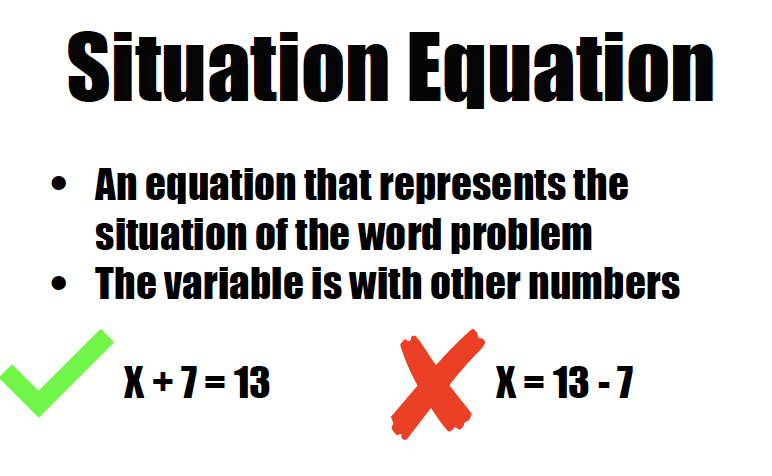Author:
Abigail
Subject:
Elementary Mathematics
Material Type:
Lesson
Level:
Upper Elementary
Tags:
• EQUATION
• Situation Equation
• Solution Equation
• uol
• uollesson
• uolmath
Language:
English
Media Formats:

# Math - Situation vs Solution Equations## Overview

Two 30 minute lesson teaching the difference between situation and solution equations in Math. Worksheet with answer key included.

Photo created by Abigail Slaugh

# Summary

This lesson will take approximately 30 minutes.

This was planned for a face-to-face classroom but can be altered to fit any.

# Background for Teachers

This lesson is about the two different types of equations that feature a variable. A variable is a letter that is a stand-in for an unknown number. You use situation and solution equations when you have a word problem with an unknown number.

A solution equation is when the variable is isolated on one side of the equation. A situation equation is when it is not. For example:

solution equation -> x = 25 • 5

situation equation -> x ÷ 5 = 25

# Step 1 - Goals and Outcomes

Learning intention: Students will be able to distinguish between situation and solution equations.

Success Criteria: Students will write situation and solution equations based on the same word problem in order to understand the differences.

# Step 2 - Planning Instruction

Student Background Knowledge:

Prior to this lesson, students will need to have an understanding of equations and variables.

Equations have an equal sign and both sides are the same.

Variables are letters that stand-in for unknown numbers.

Students also need to know use an inverse operation to solve an equation. Attached is an awesome eMedia resource on the topic.

Strategies for Diverse Learners:

One idea to support diverse learners is to give them picture vocabulary cards. This will support langauge learners or students will attention or memory deficits.

# Step 3 - Instruction

DAY 1

Hook:

Give students the following word problem:

The Lazy Panda's last soccer game was against the Angry Kittens. The crowd was made up by fan of both teams. If 2,345 people were in the cheering section and 1,678 of them were cheering for the Angry Kittens, how many people were there in support of the Lazy Pandas?

Ask the students to explain which of the following two equations relates to the above word problem:

Equation 1 -> x + 1678 = 2345

Equation 2 -> x = 2345 - 1678

Instruction:

Once the students give their reasons for or against the equations, explain to the students that that was a trick question because both equations relate to the story problem. Explain that equation 1 and 2 are different types of equations.

Equation 1 is a situation equation. Situation equations are so named because they represent the situation of the word problem. This word problem's situation is that the total of the Lazy Panda's fans (x) and the Angry Kittens' fans (1678) is 2345 fans.

Equation 2 is a solution equation. Solution equations isolate the variable and show which operation is needed to solve the word problem. In order to figure out how many fans came out in support of the Lazy Pandas, you need to subtract the number of Angry Kittens fans (1678) from the total number of fans in attendace (2345).

We Do

Display this new word problem: Margot is sick and was told to drink 32 cups of water to feel better. If she is awake for 8 hours, how many cups of water does she need to drink each hour to reach her goal?

Inform the students that you will be working together to write a situation equation and a solution equation.

Start the students off by saying for a situation equation, the 32 will be on one side of the equation. Work will students to figure out how to write the other side of the equation. Have x or w represent the number of cups Margot has to drink each hour.

Answer: 32 = x • 8

Now have the students write a solution equation. In order to do this, they have to isolate the x on one side of the equation. Remind students that equations are like balances; if you do something to one side, you have to do it to both sides. In order to get x alone, you have to divide both sides by 8. (Again, if you need a refresher on this, use the attached eMedia lesson.) This leaves you with the solution equation of x = 32 ÷ 8

Pass out the worksheet (made on Pages) and do a few with the class.

You Do

Have the students finish the rest of the worksheet on their own.

DAY 2

Students' task today is to make a Apple project that shows their understanding of this math concept. Their task is to record a song, poem, rap, instructional video, etc that explains the different between situation and solution equations. Students will primarily use iMovie for this project but they could record a presentation on Keynote. The recording needs to be at least 1 minute long with definitions and examples.

# Step 4 - Assessments

Their assessment is the complete worksheet from day 1 and the Apple project from day 2.

Attached is the rubric and example project.